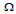# Digital Electronics - Multivibrators and 555 Timer - Discussion

### Discussion :: Multivibrators and 555 Timer - General Questions (Q.No.4)

4.

A 22-kresistor and a 0.02-F capacitor are connected in series to a 5-V source. How long will it take the capacitor to charge to 3.4 V?

 [A]. 0.44 ms [B]. 0.501 ms [C]. 0.66 ms [D]. 0.70 ms

Explanation:

No answer description available for this question.

 Sujats said: (May 2, 2011) I want to know how to solve this problem or formula for it.

 Sakshi said: (May 6, 2011) What is the formula to solve this problem.

 Korsa Taressa said: (May 13, 2011) How the answer be 0.501 ms?

 Shafeeq said: (Jul 2, 2011) Use this formula V=Vm(1-exp(-t/R*C)) Here all values are given and solve for t.

 Arvind said: (Aug 6, 2011) What is other formula without calculator for exam?

 Ambree said: (Oct 1, 2011) Wt is the formula to calculate with out calculator ?

 Vikas Kaushik said: (Jan 10, 2012) V = Vo [ {exp(-t/RC) }- 1] where V = voltage across capacitor at any time t Vo = supply voltage

 K.K said: (May 8, 2013) The charging of capacitor is calculated. T= ln(3) *RC.

 Viendong194 said: (Feb 16, 2014) Yes. this is a monostable that made by IC555. When Vc=2/3Vo , T=ln(3)*RC.

 Mohit Sharma said: (Nov 4, 2014) V(c) = V(s)[1-e((-t)/RC)]. 3.4 = 5[1-e((-t)/RC)]. 3.4/5 = [1-e((-t)/RC)]. 0.68 = 1-e((-t)/RC). e((-t)/RC) = 0.32. Taking natural log both sides, we have -t/RC = -1.139. t = 1.139 * 0.02u * 22K. t = 0.501ms.

 Aimal said: (Dec 17, 2014) t = RC*ln(Vc/Vs).

 Uday Pratap Singh said: (Oct 20, 2016) Voltage across capacitor is, Vc = V (1 - e^(-t/RC)). From question Vc = 3.4V, V = 5V, RC = 0.44 x 10^-3. Putting these values in above equation. 3.4 = 5 (1 - e^(-t/(0.44 * 10^(-3)))). After solving this equation, t = 0.501 ms.

 Harini said: (Feb 19, 2020) How this problem is solved. Are said a formula for this problem.